# Transform

Tip

## Introduction

This article explains a transformation in a point cloud context, how to transform a Zivid point cloud, and why this is a valuable feature. Lastly, it provides a tutorial with code examples.

Zivid point clouds are given relative to the Zivid camera coordinate system. Different applications for various reasons require transforming the whole point cloud or its segment, e.g., ROI or pick point, from the camera coordinate system to another coordinate system.

 Problem The Point cloud is given in the camera coordinate system, but the application requires the point cloud given in another coordinate system. Solution Transform the Zivid point cloud to another coordinate system.

## Transform API

A rigid transformation is defined by a 4×4 homogeneous transformation matrix that describes the pose between two coordinate systems. Once the transformation matrix is known, we can use Zivid SDK to transform the point cloud from the camera coordinate system to the desired coordinate system.

The following API is used to transform the point cloud:

```Zivid::PointCloud::transform(Zivid::Matrix4x4)
```
```Zivid.NET.PointCloud.Transform(float[,])
```
```Zivid.PointCloud.transform(numpy.array([4,4], dtype=numpy.float32))
```

Tip

Zivid SDK allows all affine transformations, including non-rigid ones. An example of a non-rigid transformation is scaling the point cloud by converting it from mm to m.

Transform API uses a 4x4 transformation matrix to transform a point cloud.

```const auto transformMillimetersToMeters =
Zivid::Matrix4x4{ { 0.001F, 0, 0, 0 }, { 0, 0.001F, 0, 0 }, { 0, 0, 0.001F, 0 }, { 0, 0, 0, 1 } };
```
```var transformMillimetersToMeters =
new float[,] { { 0.001F, 0, 0, 0 }, { 0, 0.001F, 0, 0 }, { 0, 0, 0.001F, 0 }, { 0, 0, 0, 1 } };
```

The transformation is done on the point cloud.

```pointCloud.transform(transformBaseToCamera);
```
```pointCloud.Transform(transformBaseToCamera);
```
```point_cloud.transform(transform_base_to_camera)
```

Note

Transform API in Zivid SDK is fast because it is done on the GPU, in parallel, while the point cloud data is still on the GPU memory. Transform implementations with third-party libraries are likely more time-consuming: CPU computations are much slower in general, and GPU computations require another copy. See Point Cloud Capture Process for more info.

Tip

• Use transform API in combination with downsample and normals APIs for performance reasons because GPU performs all these computations while the point cloud data is still on the GPU memory.

• For the fastest implementation, first, downsample the point cloud, then transform it.

• Compute normals after transforming the point cloud to have the normals in the same coordinate system as point cloud.

### Use our API to save or to load Matrices

With our API, saving or loading matrices from or to YAML files is simple, and it just takes a single line of code.

#### Save

```Zivid::Matrix4x4 zividMatrix.save("path");
```
```new Zivid.NET.Matrix4x4(zividMatrix).Save("path");
```

```Zivid::Matrix4x4 zividMatrix("path")
```
```var zividMatrix = new Zivid.NET.Matrix4x4("path");
```

## Transform in applications

This section covers applications with Zivid cameras and robots that utilize point cloud transformation. It describes typical coordinate systems in these applications and why it is helpful to transform the point cloud to these coordinate systems.

### Picking

For a robot to pick the detected object in the point cloud, its coordinates must be transformed from the camera coordinate system to a coordinate system that the robot understands.

#### Robot coordinate system

We often want to transform object coordinates or the whole point cloud to the robot base coordinate system. This is explained in detail in the article How To Use The Result Of Hand-Eye Calibration.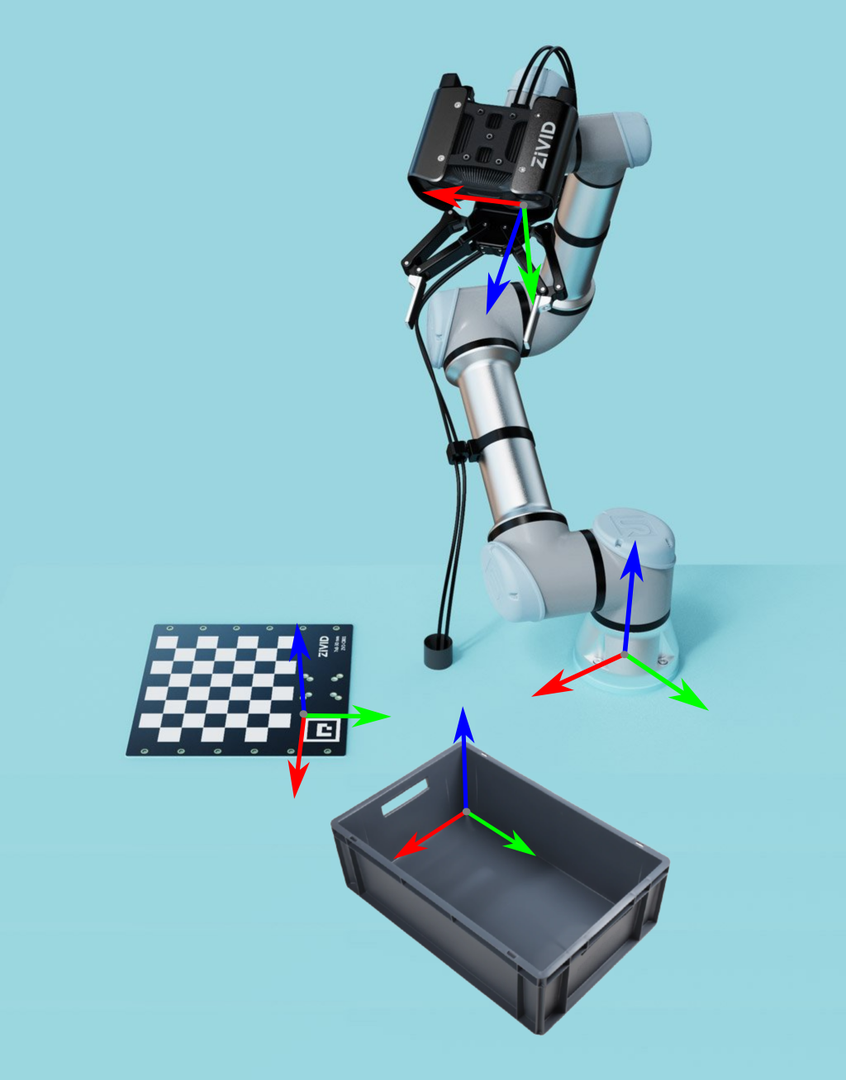#### Plane coordinate system

The camera is often not parallel with the picking surface. Transforming the point cloud so that the point cloud z-axis matches the plane z-axis helps us better understand and interpret the point cloud data. Therefore, it is common to transform the point cloud to a coordinate system on the picking surface, such as the bin floor or conveyor belt.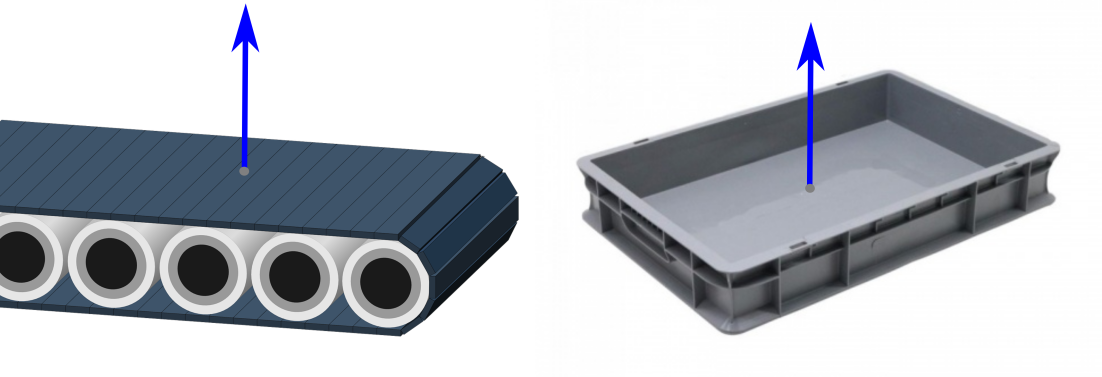#### Bin coordinate system

There is another step that can help us to better understand the point cloud data and evaluate the picking performance. That is to align the x and y axes of the point cloud with the x and y axes of the bin.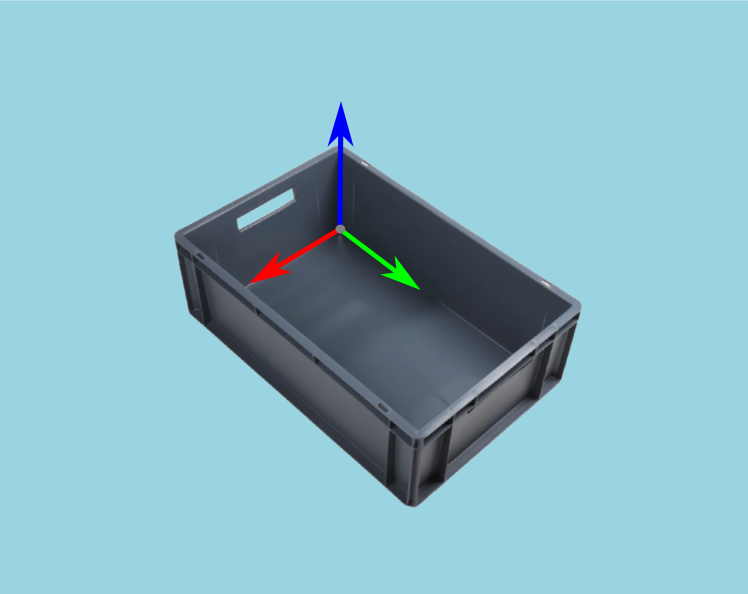Region of Interest

The camera field of view is often larger than our Region of Interest (ROI), e.g., a bin. Therefore, another reason to align bin axes with the point cloud axes is that it allows us to set a ROI box around the bin. We can then crop the point cloud based on the ROI to get only the points of the bin contents.

Tip

Smaller point clouds can make the detection faster and total picking cycle times shorter.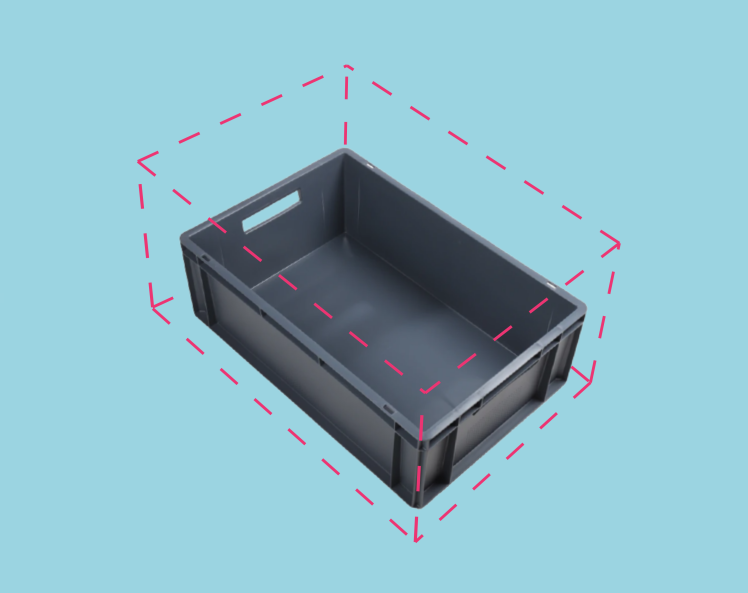For an implementation example, check out ROI Box via Checkerboard. This tutorial demonstrates how to filter the point cloud using the Zivid calibration board based on a ROI box given relative to the checkerboard.

We also have ROI Box via ArUco Marker tutorial where instead of the checkerboard we use the ArUco marker.

### Stitching

Point cloud stitching often involves transforming point clouds to a different coordinate system.

#### Multiple stationary cameras

Zivid Multi-Camera Calibration enables transforming point clouds from multiple cameras into the coordinate system of one of the cameras - the master camera. In multi-camera calibration, we often want to transform the stitched point cloud to a different coordinate system, such as the object coordinate system.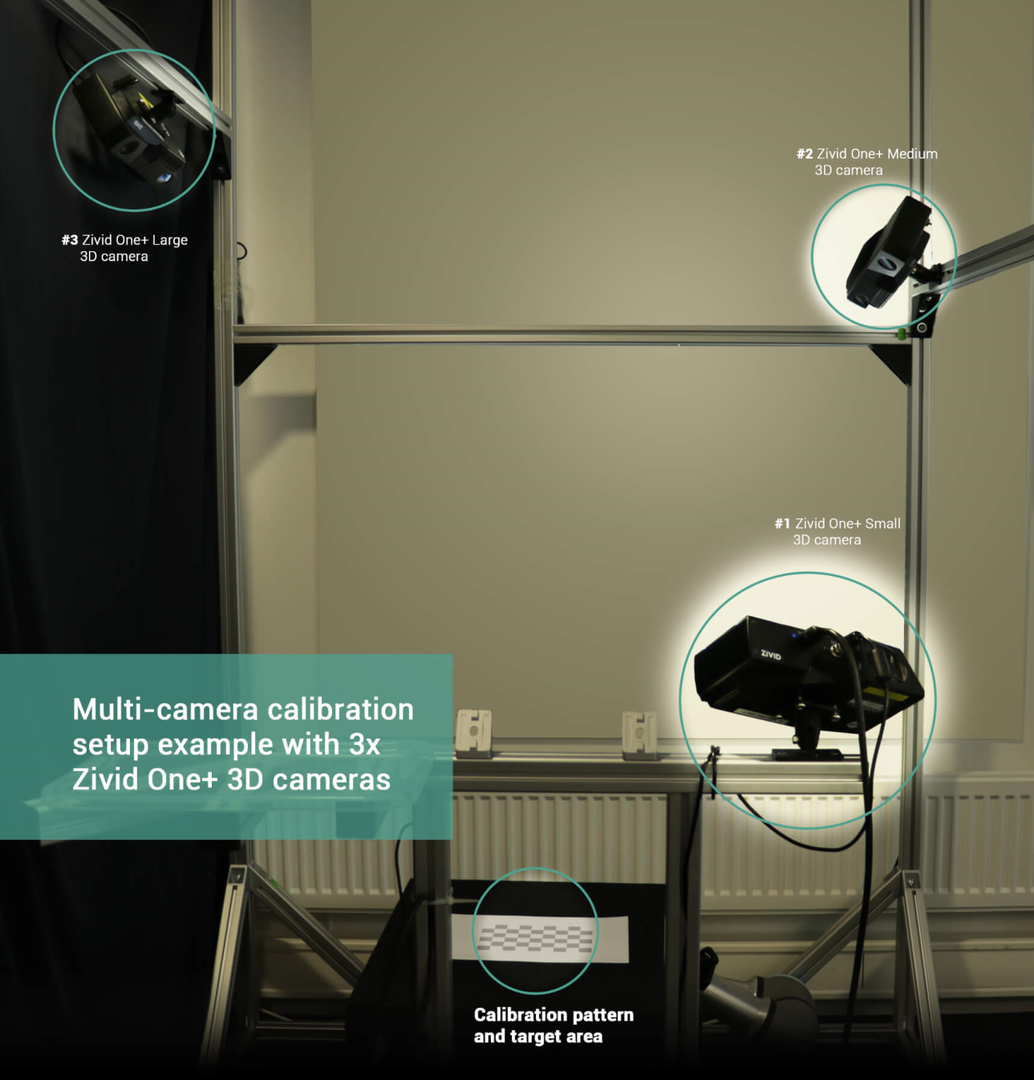#### Single robot-mounted camera

Robot-mounted camera configuration allows stitching multiple point clouds taken with the same camera from multiple views. Once the point cloud is stitched in the master camera coordinate system, it is convenient to transform it to the object coordinate system.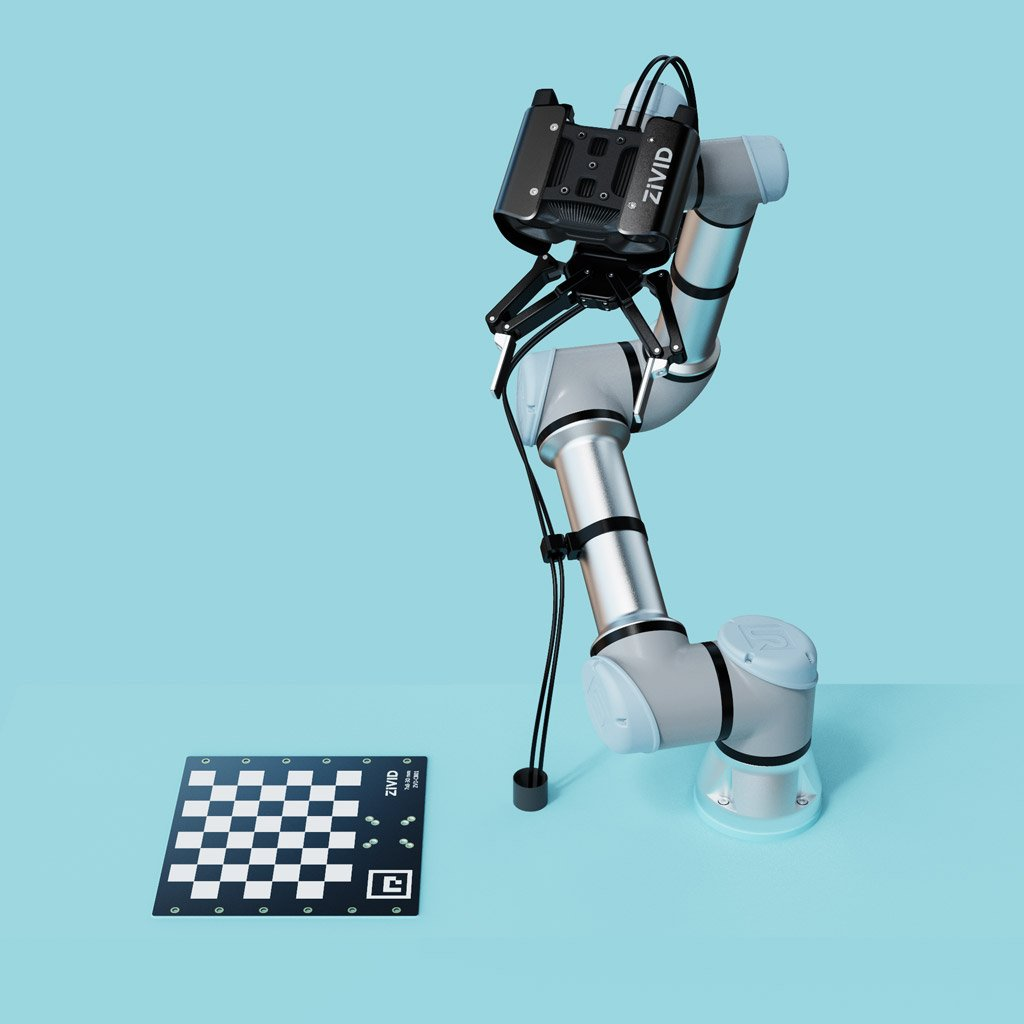#### Single camera and turntable

If we know the pose of the turntable relative to the camera, we can stitch the point clouds and transform them to the turntable coordinate system.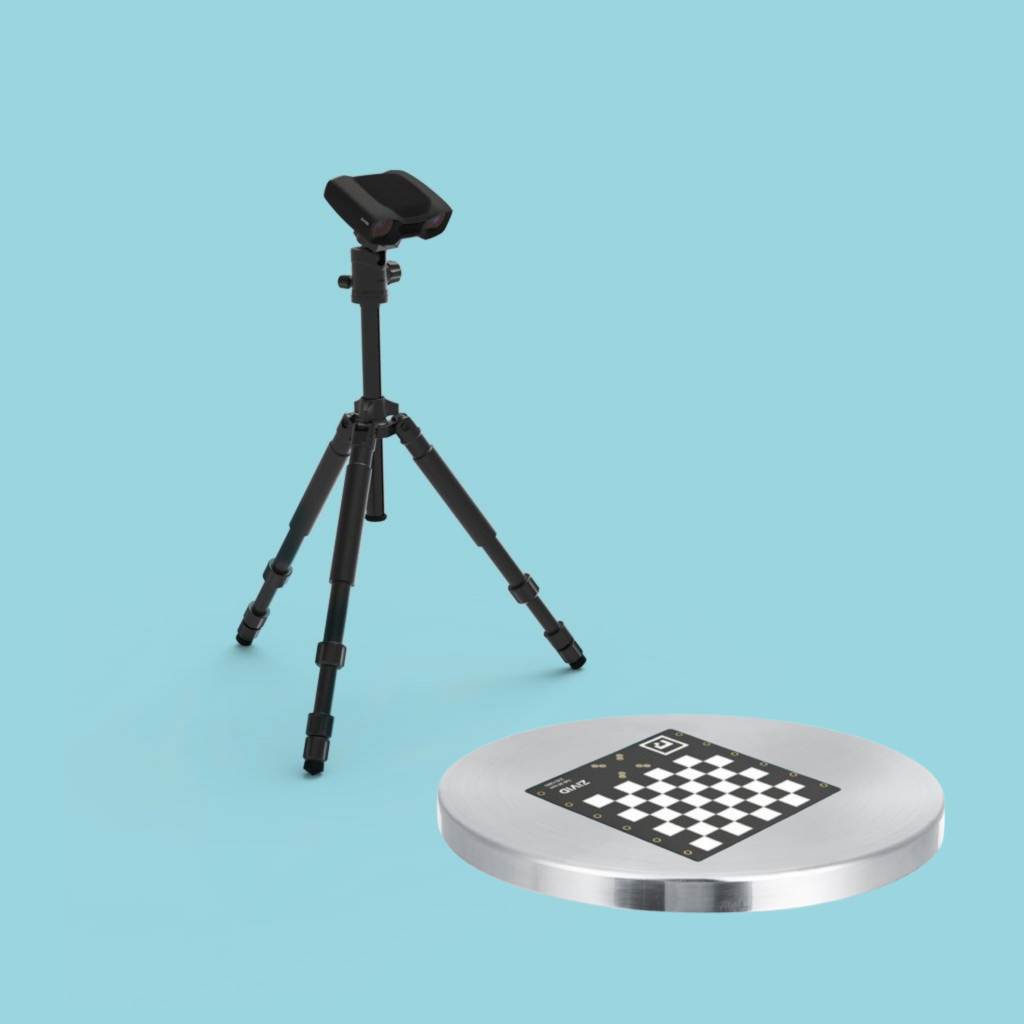Note

Multi-Camera Calibration is part of the SDK, and it uses the Zivid calibration board.

## Pose estimation

Getting the pose of the camera relative to the robot base frame is done with the Hand-Eye Calibration. If we need a pose relative to an arbitrary user-defined coordinate system, we can place helpful accessories such as fiducial markers and checkerboards in the scene. Since we know their geometry, it is relatively straightforward to estimate their pose.

For an implementation example, check out Transform via Checkerboard. This tutorial demonstrates how to estimate the pose of the checkerboard and transform a point cloud using a 4x4 homogeneous transformation matrix to the checkerboard coordinate system. We also have Transform via ArUco marker tutorial where instead of the checkerboard we use the ArUco marker.

SDK

Changes

2.0.0